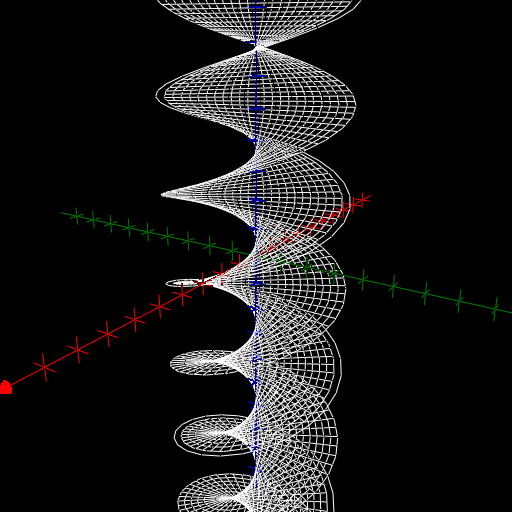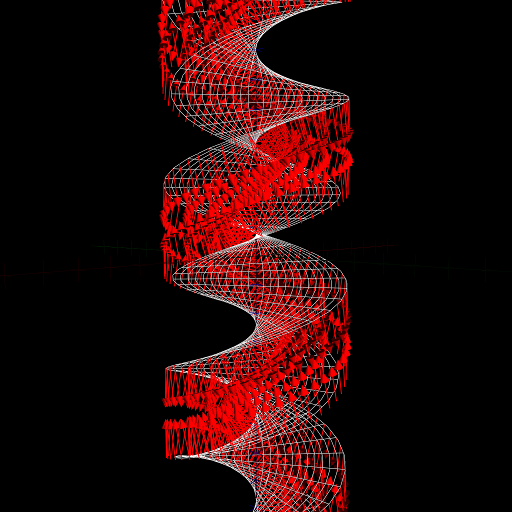$\newcommand{\sech}{\mathop{\rm sech}\nolimits} \newcommand{\csch}{\mathop{\rm csch}\nolimits} \newcommand{\R}{\mathbb{R}} \newcommand{\basis}{\underline{\mathbf{e}}}$
Current Page
Helicoid

# Helicoid

Object type: Surface

### Definition

The helicoid is the image $\mathbf{r}(\mathbb{R}^2)$ where $$\mathbf{r}(u,v) = \underline{\mathbf{e}}\begin{pmatrix}u\cos v\\u\sin v\\v\end{pmatrix}.$$ Below, the image $\mathbf{r}(\left[-\pi,\pi\right]\times \mathbb{R})$ is shown.### Properties

The following properties are with respect to the parameterisation given above.

#### Parameter-curve tangent vectors

The parameter-curve tangent vectors are $$\mathbf{r}_u(u,v) = \underline{\mathbf{e}} \begin{pmatrix}\cos v\\\sin v\\0\end{pmatrix}, \quad\quad \mathbf{r}_v(u,v) = \underline{\mathbf {e}}\begin{pmatrix}-u\sin v\\u\cos v\\1\end{pmatrix}.$$

#### Standard unit normal

The standard unit normal vector field is $$\mathbf{\hat{N}}(u,v) = \frac{1}{\sqrt{1+u^2}} \underline{\mathbf{e}}\begin{pmatrix}\sin v\\-\cos v\\u\end{pmatrix}.$$#### Area element

The area element is $$dA = \sqrt{1+u^2}~dudv.$$

#### First fundamental form

The first fundamental form of the helicoid is $$\mathcal{F}(u,v) = \begin{pmatrix}1&&0\\0&&1 + u^2\end{pmatrix}.$$

#### Second fundamental form

The second fundamental form is $$\mathcal{M}(u,v) = \frac{-1}{\sqrt{1+u^2}}\begin{pmatrix} 0&&1\\1&&0\end{pmatrix}.$$

#### Christoffel symbols

The Christoffel symbols are $$\Gamma^1_{\alpha\beta}(u,v) = \begin{pmatrix}0&&0\\0&&-u\end {pmatrix}, \quad\quad \Gamma^2_{\alpha\beta}(u,v) = \frac{u}{1+u^2}\begin{pmatrix}0&&1\\1&&0 \end{pmatrix}.$$

#### Curvatures

The principal curvatures are $$\kappa_1 = \frac{1}{1+u^2}, \quad\quad \kappa_2 = \frac{-1}{1+u^2}$$ with the corresponding principal directions $(1,0)$ and $(0,1)$. Therefore, the Gaussian and mean curvatures are $$K = \frac{-1}{\left( 1+u^2\right)^2}, \quad\quad H = 0.$$ Thus, the helicoid is a minimal surface (that is, a surface of zero mean curvature).

### Examples

Consider a (thread-like) bar of length $L > 0$ attached at its centre to a motor and used as a propeller on a vehicle. The vehicle is moving with unit speed along the $z$ axis and the propeller is perpendicular to this axis, with its centre at the origin at the origin of time. If the propeller is rotating in the positive sense (with respect to the velocity of the vehicle) with unit angular frequency, then the surface in space traced out by the propeller from the origin of time to time $t_0$ is $\mathbf{r}(\left[-L,L\right]\times\left [0,t_0\right])$.

The image of $$(u,v) \mapsto \underline{\mathbf{e}}\begin{pmatrix}au\cos v\\bu\sin v\\v\end{pmatrix}$$ is naturally called an elliptic helicoid. If $a = b$ this reduces to the standard helicoid (scaled the same amount in the $x$ and $y$ directions).